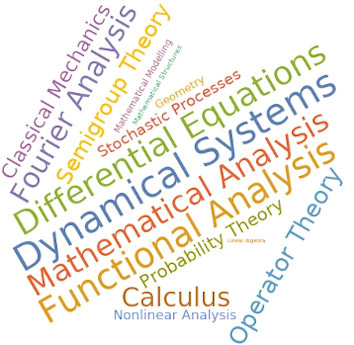# Dr Dmitri Finkelshtein

Associate Professor, Mathematics

### Telephone number

+44 (0) 1792 606291

Office - 325
Third Floor
Computational Foundry
Bay Campus

I am an Associate Professor in the Department of Mathematics at Swansea University. My research is mainly about the topics in analysis, probability, mathematical physics, and PDE inspired by and used in the theory of complex systems. I have dealt with infinite-dimensional analysis, applications of methods of functional analysis and probability theory to mathematical physics, random measures, stochastic dynamics, and mathematical biology. I am actively working on the study of non-local evolution equations arising as scaling limits for stochastic dynamics of the considered complex systems.

## Degrees

PhD 2004 & DSc (Habilitation) 2014 — Institute of Mathematics, Kyiv, Ukraine
BSc (Hons) Mathematics 1999 & MSc (Hons) Mathematics 2000 — National University of Kyiv, Ukraine

## Areas Of Expertise

• Complex Systems
• Infinite-Dimensional Analysis
• Point Processes
• Non-local Evolution Equations
• Population Ecology
• Umbral Calculus

## Career Highlights

Teaching Interests• Mathematical Analysis, Functional Analysis, Fourier Analysis
• Dynamical Systems, Ordinary and Partial Differential Equations
• Probability Theory, Stochastic Processes, Markov Chains
Research Award Highlights Collaborations